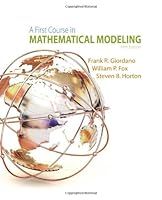# A First Course in Mathematical Modeling, 5th Edition## Book Description

Offering a solid introduction to the entire modeling process, A FIRST COURSE IN MATHEMATICAL MODELING, 5th Edition delivers an excellent balance of theory and practice, and gives you relevant, hands-on experience developing and sharpening your modeling skills. Throughout, the book emphasizes key facets of modeling, including creative and empirical model , model analysis, and model research, and provides myriad opportunities for practice. The authors apply a proven six-step problem-solving process to enhance your problem-solving capabilities -- whatever your level. In addition, rather than simply emphasizing the calculation step, the authors first help you learn how to identify problems, construct or select models, and figure out what data needs to be collected. By involving you in the mathematical process as early as possible -- beginning with short projects -- this text facilitates your progressive development and confidence in mathematics and modeling.

Chapter 1: Modeling Change
Chapter 2: The Modeling Process, Proportionality, and Geometric Similarity
Chapter 3: Model Fitting
Chapter 4: Experimental Modeling
Chapter 5: Simulation Modeling
Chapter 6: Discrete Probabilistic Modeling
Chapter 7: Optimization of Discrete Models
Chapter 8: Modeling Using Theory
Chapter 9: Modeling with Decision Theory
Chapter 10: Game Theory
Chapter 11: Modeling with a Differential Equation
Chapter 12: Modeling with of Differential Equations
Chapter 13: Optimization of Continuous Models
Chapter 14: Dimensional Analysis and Similitude
Chapter 15: of as Models

Appendix A: Problems from the Mathematics Contest in Modeling, 1985-2012
Appendix B: An Elevator Simulation Algorithm
Appendix C: The Revised Simplex Method
Appendix D: Brief Review of Techniques